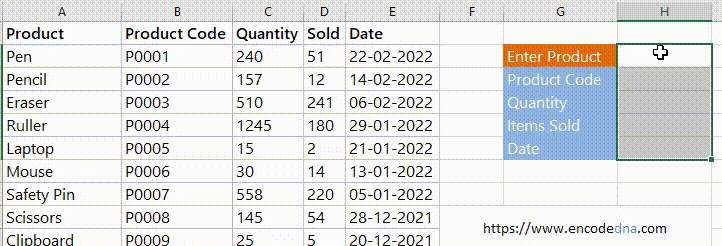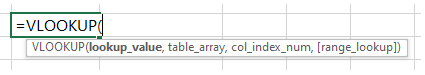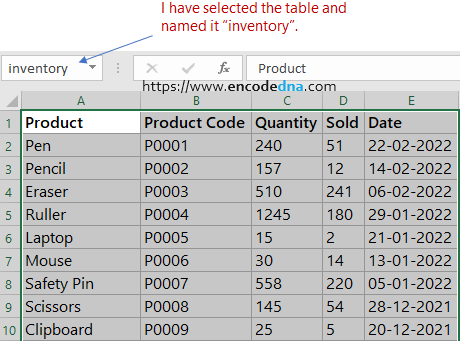﻿ How to use VLOOKUP function in VBA

# How to use VLOOKUP function in VBA

You can use VLOOKUP in Excel to search a particular value in a dataset like a named range. Even though its a worksheet function, you can use VLOOKUP in VBA for more dynamic use.I have kept the example simple and short.

I have explained about VLOOKUP in detail here in this tutorial.

Here's how a VLOOKUP function looks in an Excel worksheet.In VBA you can access VLOOKUP function through Application.WorksheetFunction and its arguments remain the same. For example,

Application.WorksheetFunction.VLookup(arg1, arg2, arg3, arg4)

Ok, now let's see the example.

Let us assume, I have data (a small inventory) in tabular format. I have named the table as "inventory" (a named range).I am assuming you know how to create named range in Excel. If you don't know, see this tutorial.

In columns H, I'll enter "name of a product" (any product from the range) and press the "Enter" key. It will call a function (a macro), which uses the "VLOOKUP" function to search for the entered product and will "return" all related data. The data will then be displayed.

You may also like... How to get live Stock market price (Stock Quotes) in your Excel worksheet?

The Macro

Open VBA editor. From "project explorer", double click the sheet where you want to write the code.

```Option Explicit

Private Sub Worksheet_Change(ByVal Target As Range)
vLookUp_Example (Target.Value)
End If
End Sub

Function vLookUp_Example(ByVal sprod As String)
On Error Resume Next

Dim sDetail As String
Dim iCols, iCnt As Integer
iCols = Range("inventory").Columns.Count       ' get total columns

For iCnt = 2 To iCols
sDetail = Application.WorksheetFunction.VLookup(sprod, Range("inventory"), iCnt, False)
sDetail = IIf(iCnt = 5, CDate(sDetail), sDetail)    ' convert string into date, since the last column has date.

Sheet3.Cells(iCnt + 1, 8) = sDetail        ' show other details
Next iCnt
End Function```

When value in "H2" changes, that is, when you enter the product name in cell H2, VBA will capture the change event and check if any change occured in H2. If true, it will call a function "vLookUp_Example". The function is a User Defined Function and it takes a parameter (an argument) that is, the product name.

I need to iterate through all the columns, because the 3rd argument in VLOOKUP is the "Column Index".

And, since the last column has Date, I am using a built-in function called CDate() to convert string into date format.

That's how you automate your Excel worksheet using VLOOKUP and VBA. 🙂